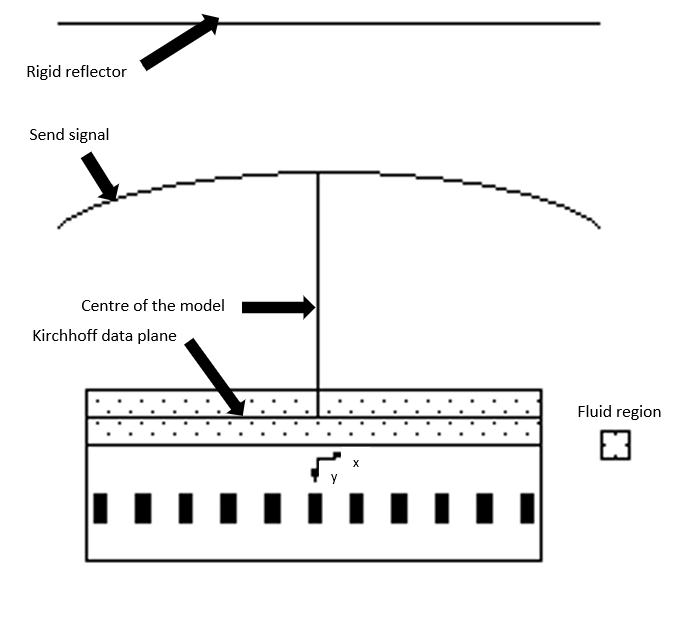# Pulse Echo Response

OnScale simulates pulse echo response by using the echo command. This command uses a similar process to extrapolation to simulate the response a transducer would generate if it were operating in a linear, isotropic transmission load with an infinite, perfect reflector at some designated point in the field.  Pulse Echo response can be implemented in both 2D and 3D models. The best approach is to break the problem into separate transmit and receive calculations. Both use basically the same physical model but with different boundary conditions and other command options. The transmit model computes the return signal at the top of the model. The receive model uses the computed return signal as an applied boundary condition on the top surface of the model and computes the model's response to the signal.To compute the return signal during the transmit calculation, the user must define a Kirchhoff data plane spanning the entire model, using the echo command. The echo option automatically computes the return signal using Kirchhoff extrapolation during the transmit calculation and saves a free-field boundary file (.flxffl), which drives the receive calculation.

The following approximations are implicit in pulse/echo analysis:

1. The computed return signal is the signal that would occur at the centerline of the model, directly above the driven transducer. It is assumed to be a normally incident plane wave at the top of the model. This assumption requires that the radius of curvature of the wave front of the return signal be large enough that a plane wave assumption is acceptable, and the reflector is far enough away in the far-field.

2. The pressure outside the region spanned by the Kirchhoff data plane is assumed to be zero. Therefore, for an accurate evaluation of the return signal, the model should be wide enough for this approximation to be valid.

3. For 2D plane strain models of the transducer region, the computed return signal maintains the 2D plane strain approximation. This results in less attenuation of the signal than if 3D effects were included.

## Transmit Model

The transmit model calculates the reflected signal as it is executing, to do this the following should be included in the model:

1. Use the echo command to define a Kirchhoff data plane in the acoustic medium, the reflector location, and other necessary parameters.

```symb reflector_dist = 200e-3                /* Reflector distance
echo
node \$i1 \$i2-1 \$j5+5 \$j5+5 1 1 1       /* Nodal range of echo plane
rcrd 0 \$reflector_dist 0. refl         /* Location of reflector
puls \$simtime                          /* Length of dataset
symm half                              /* Model symmetry     end                              ```

3. The Kirchhoff data plane should be located close to the fluid/solid interface to avoid the escape of significant energy through the sides of the model below the data plane. We recommend placing the data plane within a few elements of the fluid/solid interface. Also, there should be enough fluid elements above the Kirchhoff data plane that absorbing boundary approximations are not significant at the location of the data plane. We recommend at least one wavelength of fluid from the data plane to the top of the model for the frequencies of interest.

4. Save the time history of the echo return pressure for later plotting. The p out command group below is used to request this time history. The echo data array contains the last computed value of the extrapolated pressure.

```pout
hist echo    end```

Note that the echo time history saved to the time history (.flxhst) file is stored on the file with the same time-record information as all other time histories requested for the model. Thus the echo return pressure is time-shifted from its actual arrival by the time it would take for a wave to travel from the Kirchhoff data plane to the rigid reflector and back to the top of the model. The value of this time shift is printed in the jobs output (.flxprt) file. It can also be assigned to a symbol variable with the following symbol statement:

`symb #get { timeshft } echotime`

where the variable "timeshift" contains the value of the time shift.

Use the same model as for the transmit simulation or a reduced model containing fewer transducer elements. The model must have the same top boundary location and same grid spacing in the vicinity of the top boundary. It need include only enough of the horizontal expanse of the transducer array to capture the periodic nature of the array. This is because the return signal is assumed to be a uniform, normally incident plane wave.

For the receive simulation, make the following changes to the transmit model:

1. Remove any echo and extr command groups.

2. Use the ffld (free-field) command group to identify the freefield boundary file

`ffld    file PulseEcho.flxffl           /* Free field filename    in stnd * * -x nchk             /* Specify local axis    end`

3. Change the boundary condition for where the free field data is to ffld.

`boun    ymax ffld auto                  /* Apply free field data to the chosen boundary               end`

4. Remove any time history request for the echo data array, as it does not exist in the receive calculation.

5. Deactivate the driving voltage used in the transmit model.

6. Assign receive circuits to electrodes if different from transmit circuits.